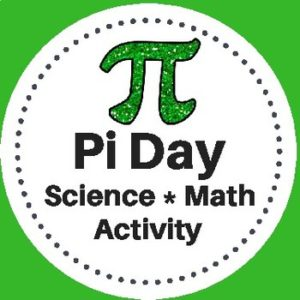Showing all 5 results

•\$3.00

This Pi Day Activity has been designed for Science and Math classrooms. Students will use measuring tools to determine the radius, diameter, and circumference of various circular objects. They will then use the data that they collect to calculate pi.

*This activity gives students the opportunity to clearly understand the concept of the number pi and practice math skills through a hands-on activity with a variety of circular objects.

•\$3.00
Here are 3 colorful “Geometry Gem” posters to display in your classroom or to place in a math, learning center as a reference.
See description below for a list of what is included!
•\$1.00

This resource, Types of Angles – Classroom Poster Set, includes 4 posters for your Math classroom…

1. Obtuse Angle
2. Acute Angle
3. Straight Angle
4. Right Angle

Print and for years of use, laminate!

•\$1.25

Here are three Math Polygon Family Tree posters to display on a bulletin board or you your Math centers. These will be a great visual addition to help introduce the relationships between shapes and shape vocabulary.

Included:

• Poster #1 Polygons will show: triangle pentagon, heptagon, quadrilateral, hexagon, octagon,
• Poster #2 Triangles will show: acute (scalene, isosceles, equilateral), obtuse (scalene, isosceles), right (scalene, isosceles)
• Poster #3 Quadrilaterals will show parallelograms (rhombuses, rectangles, squares), non-parallelograms (trapezoids, trapeziums, kites)
•\$9.99

A step by step Geometry curriculum to teach students with ease. Students will learn about angles, lines, triangles, polygons,quadrilaterals, prisms, coordinate geometry, slope and more!